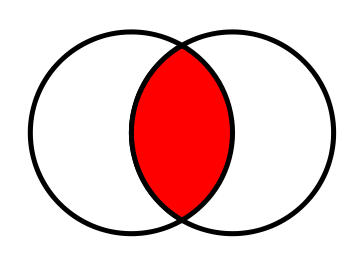# INTERSECT – the Underestimated Two-Way IN Predicate

Have you ever wondered how you could express a predicate that “feels” like the following, in SQL:
```
WHERE Var1 OR Var2 IN (1, 2, 3)

```
/u/CyBerg90 has, on reddit. The idea was to create a predicate that yields true whenever both values `Var1` and `Var2` yield either 1, 2, or 3. The canonical solution The canonical solution would obviously be to write it all out as:
```
WHERE Var1 = 1 OR Var1 = 2 OR Var1 = 3
OR    Var2 = 1 OR Var2 = 2 OR Var2 = 3

```
A lot of duplication, though. Using IN predicates Most readers would just connect the two `IN` predicates:
```
WHERE Var1 IN (1, 2, 3)
OR    Var2 IN (1, 2, 3)

```
Or the clever ones might reverse the predicates as such, to form the equivalent:
```
WHERE 1 IN (Var1, Var2)
OR    2 IN (Var1, Var2)
OR    3 IN (Var1, Var2)

```
Nicer solution using EXISTS and JOIN All of the previous solutions require syntax / expression repetition to some extent. While this may not have any significant impact performance-wise, it can definitely explode in terms of expression length. Better solutions (from that perspective) make use of the `EXISTS` predicate, constructing ad-hoc sets that are non-empty when both `Var1` and `Var2` yield either 1, 2, or 3. Here’s `EXISTS` with `JOIN`
```
WHERE EXISTS (
SELECT 1
FROM (VALUES (Var1), (Var2)) t1(v)
JOIN (VALUES (1), (2), (3)) t2(v)
ON t1.v = t2.v
)

```
This solution constructs two tables with a single value each, joining them on that value:
```+------+    +------+
| t1.v |    | t2.v |
+------+    +------+
| Var1 |    |    1 |
| Var2 |    |    2 |
+------+    |    3 |
+------+
```
Looking at a Venn Diagram, it is easy to see how JOIN will produce only those values from `t1` and `t2` that are present in both sets:Nicest solution using EXISTS and INTERSECT However, people might not think of a set intersection when they read `JOIN`. So why not make use of actual set intersection via `INTERSECT`? The following is the nicest solution in my opinion:
```
WHERE EXISTS (
SELECT v
FROM (VALUES (Var1), (Var2)) t1(v)
INTERSECT
SELECT v
FROM (VALUES (1), (2), (3)) t2(v)
)

```
Observe, how the length of the SQL statement increases with `O(m + n)` (or simply `O(N)`, where `m, n = number of values in each set`, whereas the original solutions using `IN` increase with `O(m * n)` (or simply `O(N2)`).

### INTERSECT Support in popular RDBMS

`INTERSECT` is widely supported, both in the SQL standard as well as in any of the following RDBMS that are supported by jOOQ:
• CUBRID
• DB2
• Derby
• H2
• HANA
• HSQLDB
• Informix
• Ingres
• Oracle
• PostgreSQL
• SQLite
• SQL Server
• Sybase ASE
• Sybase SQL Anywhere
In fact, the following databases also support the less commonly used `INTERSECT ALL`, which doesn’t remove duplicate values from resulting bags (see also `UNION` vs. `UNION ALL`)
• CUBRID
• PostgreSQL
Happy intersecting!

## 4 thoughts on “INTERSECT – the Underestimated Two-Way IN Predicate”

1. Why all these features never get implemented into MySQL?

2. ericjs says:

Am I suffering some massive brain fart in reading this, or did you in writing it? If you want “a predicate that yields true whenever both values Var1 and Var2 yield either 1, 2, or 3” don’t you mean AND in many of the places you wrote OR? For example shouldn’t

WHERE Var1 IN (1, 2, 3)
OR Var2 IN (1, 2, 3)

1. I suspect that this is really what the OP wanted… Only one of `Var1` or `Var2` needs to be in `(1, 2, 3)`